# Samacheer Kalvi 6th Maths Solutions Term 1 Chapter 1 Numbers Ex 1.1

## Tamilnadu Samacheer Kalvi 6th Maths Solutions Term 1 Chapter 1 Numbers Ex 1.1Question 1.
Fill in the blanks.

1. The smallest 7 digit number is _______
2. The largest 8 digit number is _______
3. The place value of 5 in 7005380 is ________
4. The expanded form of the number 76,70,905 is _______

Solution:

1. 10,00,000
2. 9,99,99,999
3. 5 × 1000 = 5000
4. 7 × 10,00,000 + 6 × 1,00,000 + 7 × 10,000 + 0 + 9 × 100 + 0 + 5 × 1
(or)
70,00,000 + 6,00,000 + 70,000 + 900 + 5

Question 2.
Say True or False.

1. In the Indian System of Numeration, the number 67999037 is written as 67999037
2. The successor of a one-digit number is always a one-digit number.
3. The predecessor of a 3-digit number is always a 3 or 4-digit number.
4. 88888 = 8 × 10000 + 8 × 100 + 8 × 10 + 8 × 1

Solution:

1. True
2. False
3. False
4. FalseQuestion 3.
Complete the given order.
Ten crore, crore, ten lakh, ____, ____, _____ , _____, _____.
Solution:
Ten crores, Crore, Ten lakh, Lakh, Ten Thousand, Thousand, Hundred, Ten, One

Question 4.
How many ten thousands are there in the smallest 6 digit number?
Solution:
Smallest six-digit number is 1,00,000
1 lakh = Ten Thousand
Another Method:
Lakh is only one place to the left of Ten thousand
1 lakh is 10 times ten thousand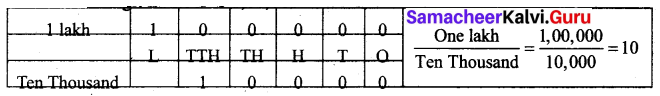1 lakh = Ten-Ten Thousand

Question 5.
Using the digits 5, 2, 0, 7, 3 forms the largest 5 digit number and the smallest 5 digit number.
Solution:
Given digits = 5, 2, 0, 7, 3
Largest 5 digit number – 75320
Smallest 5 digit number – 20357

Question 6.
Observe the commas and write down the place value of 7.

1. 56,74,56,345
2. 567,456,345

Solution:

1. 56,74,56,345
Place value of 7 is 7 × 10,00,000 = 70,00,000 = Seventy Lakhs.
2. 567,456,345
Place value of 7 is 7 × 1,000,000 = 7,000,000 = Seven Million.

Question 7.
Write the following numbers in the International system by using commas.
(i) 347056
(ii) 7345671
(iii) 634567105
(iv) 1234567890
Solution: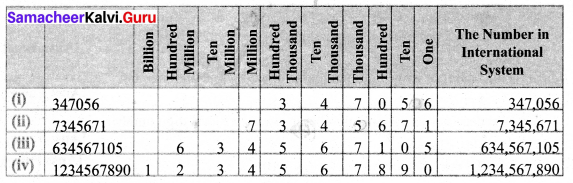Question 8.
Write the largest six-digit number and put commas in the Indian and the International Systems.
Solution:
The largest six-digit number is 999999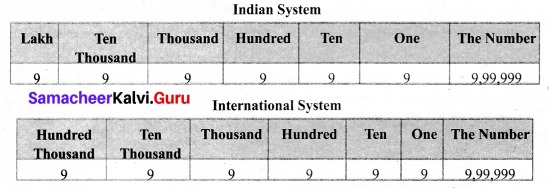Question 9.
Write the number names of the following numerals in the Indian System.
(i) 75,32,105
(ii) 9,75,63,453
Solution:
(i) 75,32,105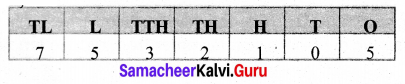Seventy-Five Lakhs Thirty-Two Thousand One Hundred and Five
(ii) 9,75,63,453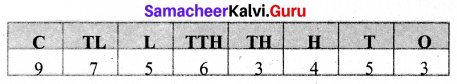Nine crores Seventy Five Lakhs Sixty Three Thousand Four Hundred and Fifty-Three.

Question 10.
Write the number names in words using the International System
(i) 345,678
(ii) 8,343,710
(iii) 103,456,789
Solution:
(i) 345,678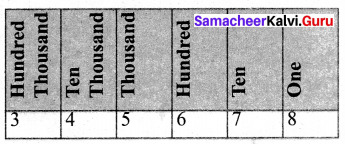Three Hundred and Forty-Five Thousand Six Hundred and Seventy-Eight
(ii) 8,343,710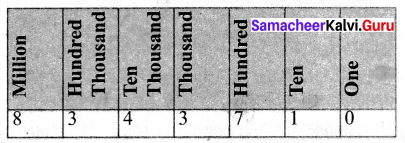Eight Million Three Hundred and Forty-Three Thousand Seven Hundred and Ten.
(iii) 103,456,789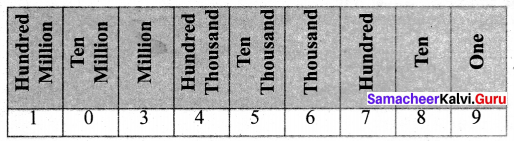One Hundred Three Million Four Hundred Fifty-Six Thousand Seven Hundred and Eighty’ Nine.Question 11.
Write the number name in numerals.

1. Two crores thirty lakhs fifty-one thousand nine hundred eighty.
2. Sixty-six million three hundred forty-five thousand twenty-seven.
3. Seven hundred eighty-nine million, two hundred thirteen thousand four hundred fifty six.

Solution:

1. 2,30,51,980
2. 66,345,027
3. 789,213,456

Question 12.
Tamil Nadu has about twenty-six thousand three hundred forty-five square kilometre of Forest land. Write the number mentioned in the statement in the Indian System.
Solution:
26,345 sq km.

Question 13.
The number of employees in the Indian Railways is about 10 lakhs. Write this in the International System of numeration.
Solution:
1.000,000 (one million)

Objective Type Questions

Question 14.
1 billion is equal to
(a) 100 crore
(b) 100 million
(c) 100 lakh
(d) 10000 lakh
Solution:
(a) 100 crore

Question 15.
The successor of 10 million is
(a) 1000001
(b) 10000001
(c) 9999999
(d) 100001
Solution:
(b) 10000001

Question 16.
The difference between successor and predecessor of 99999 is
(a) 90000
(b) 1
(c) 2
(d) 99001
Solution:
(c) 2Question 17.
The expanded form of the number 6,70,905 is
(a) 6 × 10000 + 7 × 1000 + 9 × 100 + 5 × 1
(b) 6 × 10000 + 7 × 1000 + 0 × 100 + 9 × 100 + 0 × 10 + 5 × 1
(c) 6 × 1000000 + 7 × 10000 + 0 × 1000 + 9 × 100 + 0 × 10 + 5 × 1
(d) 6 × 100000 + 7 × 10000 + 0 × 1000 + 9 × 100 + 0 × 10 + 5 × 1
Solution:
(d) 6 × 100000 + 7 × 10000 + 0 × 1000 + 9 × 100 + 0 × 10 + 5 × 1